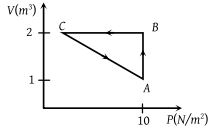# NEET Physics Thermodynamics Questions SolvedAn ideal gas is taken through the cycle ABCA, as shown in the figure. If the net heat supplied to the gas in the cycle is 5 J, the work done by the gas in the process CA is(1) – 5 J

(2) – 10 J

(3) – 15 J

(4) – 20 J

Concept Videos :-

#29 | Work done in Cyclic Process
#30 | Solved Example: 13

Concept Questions :-

Cyclic process

(1) For cyclic process. Total work done $={W}_{AB}+{W}_{BC}+{W}_{CA}$

ΔWAB = PΔV = 10(2 – 1) = 10J and ΔWBC =0

(as V = constant)

ΔQ = ΔU + ΔW

ΔU = 0 (Process ABCA is cyclic)

⇒ ΔQ = ΔWAB + ΔWBC + ΔWCA

⇒ 5 = 10 + 0 + ΔWCA ⇒ ΔWCA = – 5 J

Difficulty Level:

• 55%
• 28%
• 12%
• 8%
Crack NEET with Online Course - Free Trial (Offer Valid Till September 21, 2019)